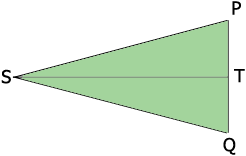SEARCH HOMEMath Central Quandaries & QueriesQuestion from Oscar: If I walked 620' and my compass was off by 8 degrees how many feet from my heading was I? Thank YouHi Oscar,

In my diagram (not to scale), $S$ is your start position, $P$ is the point where you planned to arrive and $Q$ is the point where you actually arrived. The angle $PSQ$ measures 8 degrees and $T$ is the midpoint of $PQ.$The angles $PST$ and $TSQ$ are congruent (do you see why) and hence the measure of the angle $PST$ is 4 degrees. The angle $STP$ is a right angle (do you see why). What trig function relates $|TP|, |PS|$ and the measure of the angle $PST?$

PennyMath Central is supported by the University of Regina and The Pacific Institute for the Mathematical Sciences.